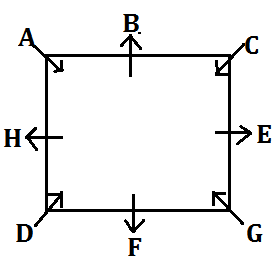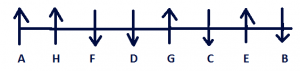Latest Banking jobs   »   Reasoning Ability Quiz For IBPS RRB...

# Reasoning Ability Quiz For IBPS RRB PO, Clerk Prelims 2021- 2nd June

Directions (1-5): Study the following information carefully and answer the given questions:
Seven boxes are placed one above other, each box has different weight. All boxes are arranged in such a manner, lightest box is placed in top of the stack and the heaviest box is placed at bottom. One of the boxes is 75kg.
More than three boxes are placed in between box F and box G. Only two boxes are placed in between D and A which is 60kg. Three boxes are placed in between B and C. The third lightest box is 35kg. Box F is lighter than D. Box C is heavier than B. Weight of box E is double of box D. Box F is 13kg lighter than box B which is 10kg heavier than D. Difference between the weight of box A and C is 21kg.

Q1. What is the weight of the Second lightest Box?
(a) 28kg
(b) 25kg
(c) 30kg
(d) 21kg
(e) 20kg

Q2. Which of the following box is heavier than B but lighter than A?
(a) C
(b) D
(c) E
(d) F
(e) G

Q3. How many boxes are lighter than D?
(a) Four
(b) Three
(c) Two
(d) One
(e) None

Q4. What is the weight of the box which is placed exactly middle in the stack?
(a) 50kg
(b) 55kg
(c) 60kg
(d) 58kg
(e) 45kg

Q5. Which of the following box is placed at the bottom of the stack?
(a) G
(b) D
(c) F
(d) A
(e) C

Directions (6-10): Study the following information carefully and answer the given questions.
Eight Persons are sitting around a square table, four of them sit at four corners facing inside while four sits at the middle of the sides facing outside. B sits third to the left of D. H sits second to the left of B and G sits third to the left of H. F is an immediate neighbour of D. A sits third to the left of the one who sits second to the left of F. E does not sits at corner. C sits immediate right of B.

Q6. How many persons sit between D and E, when counted right of D?
(a) Three
(b) One
(c) None
(d) Two
(e) Four

Q7. Who among the following sits immediate right of H?
(a) E
(b) D
(c) A
(d) C
(e) B

Q8. Who among the following faces G?
(a) D
(b) C
(c) B
(d) H
(e) A

Q9. What is the position of E with respect of B?
(a) Immediate right
(b) Second to the right
(c) Immediate Left
(d) Third to the left
(e) None of these

Q10. Four of the following are alike in a certain way so form a group, which among the following does not belong to that group?
(a) C
(b) D
(c) A
(d) B
(e) G

Direction (11-15): Study the given information carefully and answer the questions that follow:
There are eight friends A, B, C, D, E, F, G and H who are sitting in a row such that some are facing in the south direction and some are facing north direction. F and D face the same direction as B is facing. D sits third to the right of A. C and E are immediate neighbours but both face opposite directions with respect to each other. A sits at one of the corner. H sits fourth to the right of C. More than three persons sit between B and F. G sits third right of H. C is neither immediate neighbour of A nor D. E does not sit at any end. F sits immediate right of D. G is facing same direction as E who does not face south.

Q11. Who among the following sits immediate right of H?
(a) G
(b) C
(c) E
(d) F
(e)None of these

Q12. How many persons sit between D and E?
(a) Four
(b) One
(c) Three
(d) Two
(e) None

Q13. Who among the following sit immediate left of the one who sits third right of G?
(a) H
(b) F
(c) A
(d) E
(e) No one

Q14. What is the position of A with respect of F?
(a) Immediate right
(b) Third to the left
(c) Second to the right
(d) Immediate left
(e) None of these

Q15. Who among the following sits second right of B?
(a) G
(b) A
(c) F
(d) C
(e) None of these

Practice More Questions of Reasoning for Competitive Exams:

###### Study Plan for IBPS RRB PO/Clerk Prelims 2021

Solutions

Solution (1-5):
Sol.S1. Ans.(b)
S2. Ans.(c)
S3. Ans.(d)
S4. Ans.(a)
S5. Ans.(e)

Solution (6-10):
Sol.S6. Ans.(d)
S7. Ans.(c)
S8. Ans.(e)
S9. Ans.(b)
S10. Ans.(d)

Solutions (11-15):
Sol.S11. Ans.(d)
S12. Ans.(d)
S13. Ans.(e)
S14. Ans.(c)
S15. Ans.(d)